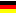Vacuum / Pressure unit conversion
 from convert into: atmosphere (atm) bar (b) hectopascal (hPa) kilogram per sq. cm (kgf/cm2) kilogram per sq. meter (kgf/m2) kilopascal (kPa) millibar millimeter of mercury (mmHg) pascal (Pa) pounds per square inch (psi) torr (Torr) atmosphere (atm) bar (b) hectopascal (hPa) kilogram per sq. cm (kgf/cm2) kilogram per sq. meter (kgf/m2) kilopascal (kPa) millibar millimeter of mercury (mmHg) pascal (Pa) pounds per square inch (psi) torr (Torr)

Pascal (Pa)

Pa=N/m² : The Pascal (Pa) is the SI unit, 1 Bar = 10E5.Pa = 101 kPa = 0.1 MPa
A pressure of 1 Pa applied on a surface A=1m² generates a force F=1N (1N=1 kg.m.s-²).

1 Torr = 4/3 mbar, =133.3 Pa

1 standard atmosphäre, 1 atm. = 760 Torr = 1013 mbar
1 tech. atmosphere, 1 At = 1kp/cm² = 981 mbar

das relative Vakuum in %: V% = (Po-P)/Po, 300mbar => (1013-300)/1013= 70% Vakuum
or P=Po-(Po.V%), 30% in Torr => 760-(760.0.3)=532 Torr

atmospheric pressure (1 Atm.)

= 1.013 bar, =1013 millibars (mbar), = 101325 Pascal (Pa); =101 kPa
= 760 millimeters of mercury absolute (mmHgA) = 760 Torr (1 Torr = 1 mm HgA)
= 29.92 inches of mercury absolute ("HgA), = 14.7 pounds per square inch absolute (lb/in², PSIA)Vakuum-/Druckeinheiten Umrechnung

all vacuum pump manufacturers

procurement (projects, enquiries)

news for the vacuum technology

atm. pressure (1 Atm.)

1.013 bar = 1013 millibars (mbar)

= 101325 Pascal (Pa), = 101 kPa

= 760 Millimeter Quecksilbersäule (mmHgA) = 760 Torr (1 Torr = 1 mm HgA)

= 29.92 inches of mercury absolute ("HgA),

= 14.7 pounds per square inch absolute (lb/in², PSIA)

Atmospheric pressure is often measured with a mercury barometer, and a height of approximately 760 millimetres (30 in) of mercury is often used to illustrate (and measure) atmospheric pressure. However, since mercury is not a substance that humans commonly come in contact with, water often provides a more intuitive way to visualize the pressure of one atmosphere:

One atmosphere (101 kPa or 14.7 psi) is the amount of pressure that can lift water approximately 10.3 m (34 ft).

10.3 m (34 ft) is also the maximum height to which a column of water can be drawn up by suction / vy a vacuum.

Pascal_(unit) in Wikipedia     Bar (unit) in Wikipedia     www.vacuum-guide.com